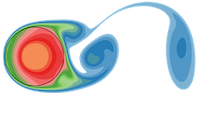FLASH-X Doxygen Generated Documentation From Interface Source Code
Grid_getCellCoords.F90 File Reference

Go to the source code of this file.

## Functions/Subroutines

subroutine Grid_getCellCoords (axis, edge, level, lo, hi, coordinates)

## ◆ Grid_getCellCoords()

 subroutine Grid_getCellCoords ( integer, intent(in) axis, integer, intent(in) edge, integer, intent(in) level, integer, dimension(1:MDIM), intent(in) lo, integer, dimension(1:MDIM), intent(in) hi, real, dimension(:), intent(out) coordinates )

This subroutine is an accessor function that gets the coordinates of the cells in a given block. Coordinates are retrieved one axis at a time, meaning you can get the i, j, _or_ k coordinates with one call. If you want all the coordinates, all axes, you need to call Grid_getCellCoords 3 times, one for each axis. The code carries coordinates at cell centers as well as faces. It is possible to get coordinates for CENTER, only LEFT_EDGE, only RIGHT_EDGE or for all FACES along a dimension.

axis - specifies the integer index coordinates of the cells being retrieved. axis can have one of three different values, IAXIS, JAXIS or KAXIS (defined in constants.h as 1,2 and 3)

edge - integer value with one of four values, LEFT_EDGE, RIGHT_EDGE, CENTER or FACES The edge argument specifies what side of the zone to get, the CENTER point, the LEFT_EDGE or the RIGHT_EDGE of the zone. FACES gets the left and right face of each cell, but since two adjacent cells have a common face, there are only N+1 unique values if N is the number of cells.

level - refinement level. This is 1-based, i.e., the root level is numbered 1.

lo - Indices of a low point, in the level-wide integer index space. Only the component indicated by the axis argument is used. The coordinates returned pertain to cells in the range lo(axis) ... hi(axis).

hi - Indices of a high point, in the level-wide integer index space. Only the component indicated by the axis argument is used. The coordinates returned pertain to cells in the range lo(axis) ... hi(axis).

coordinates - The array holding the data returning the coordinate values. The array must be at large enough to hold the number of coordinate values that are requested occording to lo and hi arguments. That is, the following must be true:

if edge = CENTER/LEFT_EDGE/RIGHT_EDGE then size(coordinates) >= hi(axis) - lo(axis) + 1 ;

If edge=FACES then size(coordinates) >= hi(axis) - lo(axis) + 2 .

EXAMPLE

1. Getting cell centered values

#include "constants.h"

integer :: coordSize integer,allocatable :: xCoord(:) integer :: level,lo(3),hi(3) .....

do while(itorisValid()) call itorcurrentTile(tileDesc) level = tileDesc % level lo = tileDesc % limits(LOW ,:) hi = tileDesc % limits(HIGH,:)

coordSize = hi(IAXIS) - lo(IAXIS) + 1 allocate(xCoord(coordSize)) !sized to be number of coords returned

call Grid_getCellCoords(IAXIS, CENTER, level, lo, hi, xCoord) ..... deallocate(xCoord) call itornext() end do

2. Getting face values

#include "constants.h"

integer :: coordSize integer,allocatable :: xCoord(:) integer :: level,lo(3),hi(3)

do while(itorisValid()) call itorcurrentTile(tileDesc) level = tileDesc % level lo = tileDesc % limits(LOW ,:) hi = tileDesc % limits(HIGH,:)

coordSize = hi(IAXIS) - lo(IAXIS) + 2 allocate(xCoord(coordSize)) !sized to be number of coords returned

call Grid_getCellCoords(IAXIS, FACES, level, lo, hi, xCoord) ..... deallocate(xCoord) call itornext() end do

Definition at line 129 of file Grid_getCellCoords.F90.